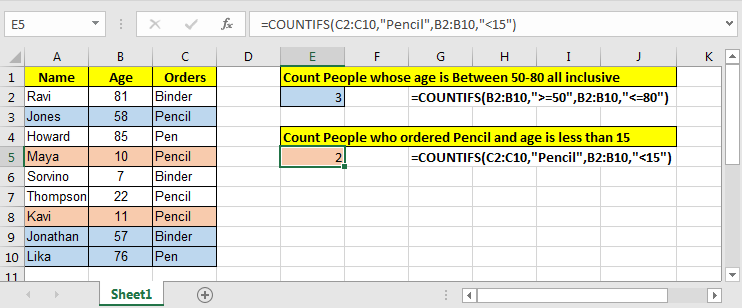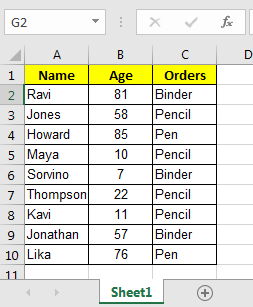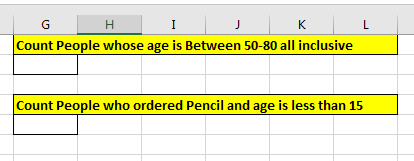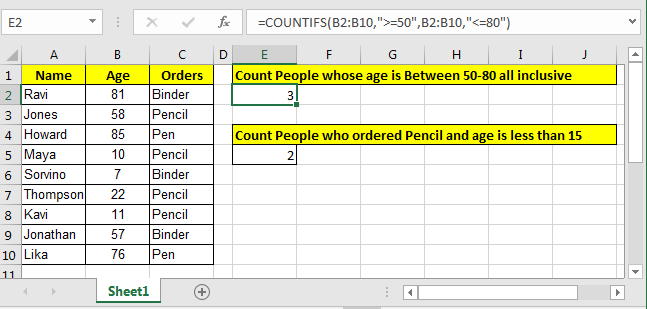# COUNTIFS Two Criteria Match

We want to count something in range only if two conditions match in excel. Here we can use Excel’s COUNTIFS function. COUNTIFS function can count on multiple criteria. And if all criteria is matched only then a row is counted. It kind of AND logic. So lets get started…Generic Formula

=COUNTIFS(criteria_range1, criteria1, criteria_range2, criteria2)

Criteria_range1: This is the first criteria range. This is mandatory. Your first condition for will be from this range.
Criteria1: This is the first criteria from criteria range1.

Criteria_range2: This is the second criteria range for your second condition. This can be the same as the first one.
Criteria2: The criteria2 is the second condition from criteria range 2.
Now, let's have an example:

Example
We have this data for our COUNTIFS example.And, we have these two queries to answer.So, for the first query we have two conditions from one single column, Age.
To answer the query, Count People whose age is Between 50-80 all-inclusive, write this COUNTIFS formula.

=COUNTIFS(B2:B10,">=50",B2:B10,"<=80")

Note that we have the same criteria range B2:B10 for both conditions. Excel has no objection at the use of the same criteria ranges. With the picked data output would be 3.

How does it work?
Simple, the k function will first look for all values in range B2:B10 that will be {81,58,85,57,76}. Then in {81,58,85,57,76} list it will look all values that are less than or equal to 80 and that will be {58,57,76}. And that is 3.
Now in the second query in which need to Count People who ordered Pencil and age is less than 15. We have to count on multiple criteria and different columns.
So two answer this query write this COUNTIFS formula.

=COUNTIFS(C2:C10,"Pencil",B2:B10,"<15")

Note that we used two different columns for our condition.Well this is the classic way of counting in excel for two or more criteria. But there is an alternative way to count if two criteria match.

An alternative way of COUNTIFS to COUNT If there are Two Criteria to Match
So lets take the same example and queries as above.
Count People whose age is Between 50-80 all-inclusive

=SUMPRODUCT((B2:B10>=50)*(B2:B10<=80))

Yes, SUMPRODUCT function can count too. I have explained SUMPRODUCT function in detail here.
Count People who ordered Pencil and age is less than 15

=SUMPRODUCT((C2:C10="Pencil")*(B2:B10<15))

Basically, the above SUMPRODUCT formula will behave as COUNTIFS functions and if would write a generic formula for this, it would look like…
Generic Formula to count on two conditions using SUMPRODUCT function

=SUMPRODUCT((range1(criteria1))*(range2(criteria2)))

Here criteria1 and criteria2 can any be condition that would generate an array of True and False from range1 and range2. Eg. =”abc”, >20, <>”2x”, ISNUMBER(range), etc.

Ok, so I covered here how to use COUNTIFS function when you have two criteria. But if still, it wasn’t the solution you were looking for, let me know your scenario in the comments section below.

Related Articles:

COUNTIFS With OR For Multiple Criteria

Count Cells That Contain This Or That

How To Count Unique Text in Excel

Popular Articles:

50 Excel Shortcuts to Increase Your Productivity

The VLOOKUP Function in Excel

COUNTIF in Excel 2016

How to Use SUMIF Function in Excel

Terms and Conditions of use

The applications/code on this site are distributed as is and without warranties or liability. In no event shall the owner of the copyrights, or the authors of the applications/code be liable for any loss of profit, any problems or any damage resulting from the use or evaluation of the applications/code.